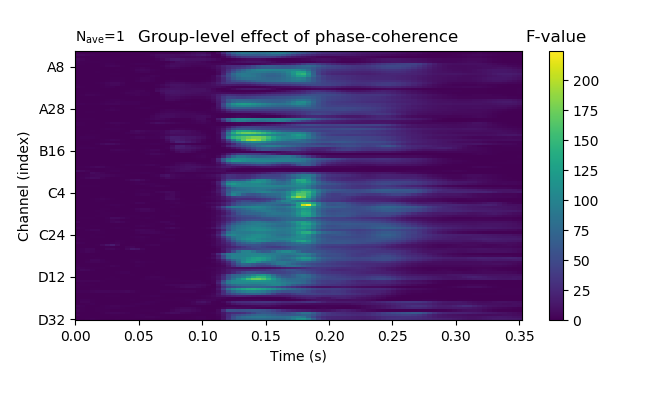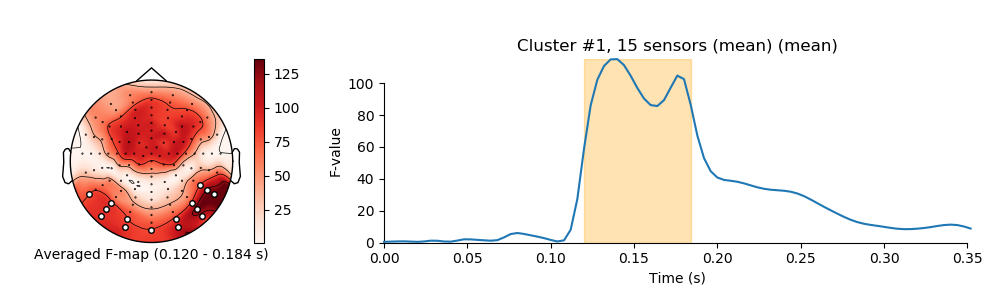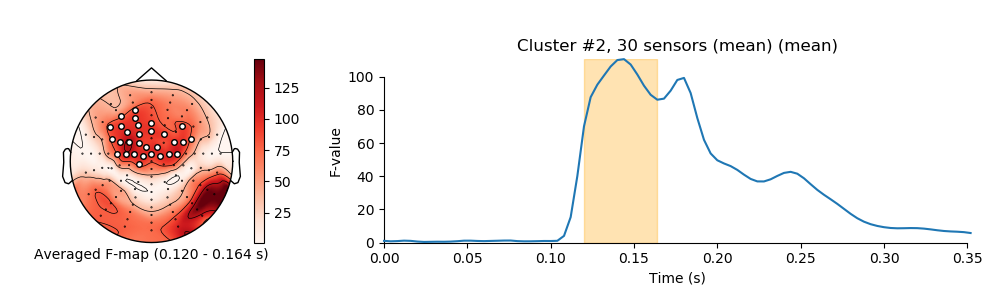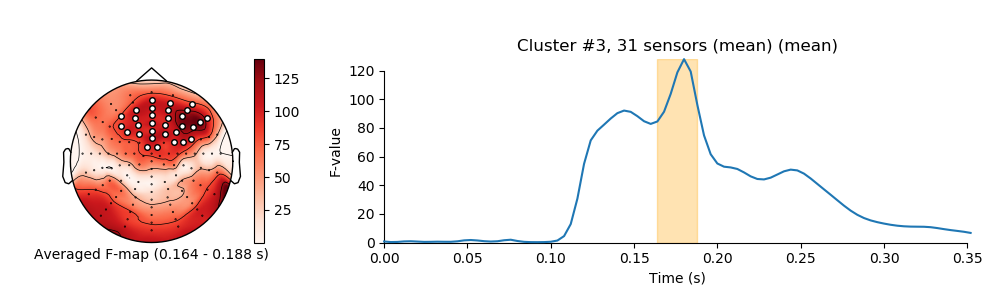# Plot spatiotemporal clustering results for effect of continuous variable¶

```# Authors: Jose C. Garcia Alanis <alanis.jcg@gmail.com>
#

import numpy as np
import matplotlib.pyplot as plt

from mpl_toolkits.axes_grid1 import make_axes_locatable

from sklearn.linear_model import LinearRegression

from mne.stats.cluster_level import _setup_connectivity, _find_clusters, \
_reshape_clusters
from mne.channels import find_ch_connectivity
from mne.datasets import limo
from mne.decoding import Vectorizer, get_coef
from mne.evoked import EvokedArray
from mne.viz import plot_topomap, plot_compare_evokeds, tight_layout
from mne import combine_evoked, find_layout
```

Here, we’ll import multiple subjects from the LIMO-dataset and compute group-level beta-coefficients for a continuous predictor, in addition we show how confidence (or significance) levels can be computed for this effects using the bootstrap-t technique and spatiotemporal clustering

```# list with subjects ids that should be imported
subjects = list(range(1, 19))
# create a dictionary containing participants data for easy slicing
limo_epochs = {str(subj): limo.load_data(subject=subj) for subj in subjects}

# interpolate missing channels
for subject in limo_epochs.values():
subject = subject.crop(tmin=0, tmax=0.35)
# only keep eeg channels
subject.pick_types(eeg=True)
```

Out:

```1055 matching events found
No baseline correction applied
0 projection items activated
1052 matching events found
No baseline correction applied
0 projection items activated
1072 matching events found
No baseline correction applied
0 projection items activated
1050 matching events found
No baseline correction applied
0 projection items activated
1118 matching events found
No baseline correction applied
0 projection items activated
1108 matching events found
No baseline correction applied
0 projection items activated
1060 matching events found
No baseline correction applied
0 projection items activated
1030 matching events found
No baseline correction applied
0 projection items activated
1059 matching events found
No baseline correction applied
0 projection items activated
1038 matching events found
No baseline correction applied
0 projection items activated
1029 matching events found
No baseline correction applied
0 projection items activated
943 matching events found
No baseline correction applied
0 projection items activated
1108 matching events found
No baseline correction applied
0 projection items activated
998 matching events found
No baseline correction applied
0 projection items activated
1076 matching events found
No baseline correction applied
0 projection items activated
1061 matching events found
No baseline correction applied
0 projection items activated
1098 matching events found
No baseline correction applied
0 projection items activated
1103 matching events found
No baseline correction applied
0 projection items activated
Computing interpolation matrix from 113 sensor positions
Interpolating 15 sensors
Computing interpolation matrix from 117 sensor positions
Interpolating 11 sensors
Computing interpolation matrix from 121 sensor positions
Interpolating 7 sensors
Computing interpolation matrix from 119 sensor positions
Interpolating 9 sensors
Computing interpolation matrix from 122 sensor positions
Interpolating 6 sensors
Computing interpolation matrix from 118 sensor positions
Interpolating 10 sensors
Computing interpolation matrix from 117 sensor positions
Interpolating 11 sensors
Computing interpolation matrix from 117 sensor positions
Interpolating 11 sensors
Computing interpolation matrix from 121 sensor positions
Interpolating 7 sensors
Computing interpolation matrix from 116 sensor positions
Interpolating 12 sensors
/home/josealanis/Documents/github/mne-stats/examples/group_level/plot_spatiotemporal_cluster.py:41: RuntimeWarning: No bad channels to interpolate. Doing nothing...
Computing interpolation matrix from 115 sensor positions
Interpolating 13 sensors
Computing interpolation matrix from 122 sensor positions
Interpolating 6 sensors
Computing interpolation matrix from 114 sensor positions
Interpolating 14 sensors
Computing interpolation matrix from 117 sensor positions
Interpolating 11 sensors
Computing interpolation matrix from 125 sensor positions
Interpolating 3 sensors
Computing interpolation matrix from 126 sensor positions
Interpolating 2 sensors
Computing interpolation matrix from 122 sensor positions
Interpolating 6 sensors
```

regression parameters

```# variables to be used in the analysis (i.e., predictors)
predictors = ['intercept', 'face a - face b', 'phase-coherence']

# number of predictors
n_predictors = len(predictors)

# save epochs information (needed for creating a homologous
# epochs object containing linear regression result)
epochs_info = limo_epochs[str(subjects)].info

# number of channels and number of time points in each epoch
# we'll use this information later to bring the results of the
# the linear regression algorithm into an eeg-like format
# (i.e., channels x times points)
n_channels = len(epochs_info['ch_names'])
n_times = len(limo_epochs[str(subjects)].times)

# also save times first time-point in data
times = limo_epochs[str(subjects)].times
tmin = limo_epochs[str(subjects)].tmin
```

create empty objects for the storage of results

```# place holders for bootstrap samples
betas = np.zeros((len(limo_epochs.values()),
n_channels * n_times))

# dicts for results evoked-objects
betas_evoked = dict()
t_evokeds = dict()
```

run regression analysis for each subject

```# loop through subjects, set up and fit linear model
for iteration, subject in enumerate(limo_epochs.values()):

# --- 1) create design matrix ---

# add intercept (constant) to design matrix
design = design.assign(intercept=1)

# effect code contrast for categorical variable (i.e., condition a vs. b)
design['face a - face b'] = np.where(design['face'] == 'A', 1, -1)

# order columns of design matrix
design = design[predictors]

# column of betas array (i.e., predictor) to run bootstrap on
pred_col = predictors.index('phase-coherence')

# --- 2) vectorize (eeg-channel) data for linear regression analysis ---
# data to be analysed
data = subject.get_data()

# vectorize data across channels
Y = Vectorizer().fit_transform(data)

# --- 3) fit linear model with sklearn's LinearRegression ---
# we already have an intercept column in the design matrix,
# thus we'll call LinearRegression with fit_intercept=False
linear_model = LinearRegression(fit_intercept=False)
linear_model.fit(design, Y)

# --- 4) extract the resulting coefficients (i.e., betas) ---
# extract betas
coefs = get_coef(linear_model, 'coef_')
# only keep relevant predictor
betas[iteration, :] = coefs[:, pred_col]

# the matrix of coefficients has a shape of number of observations in
# the vertorized channel data by number of predictors;
# thus, we can loop through the columns i.e., the predictors)
# of the coefficient matrix and extract coefficients for each predictor
# in order to project them back to a channels x time points space.
lm_betas = dict()

# extract coefficients
beta = betas[iteration, :]
# back projection to channels x time points
beta = beta.reshape((n_channels, n_times))
# create evoked object containing the back projected coefficients
lm_betas['phase-coherence'] = EvokedArray(beta, epochs_info, tmin)

# save results
betas_evoked[str(subjects[iteration])] = lm_betas

# clean up
del linear_model
```

compute mean beta-coefficient for predictor phase-coherence

```# subject ids
subjects = [str(subj) for subj in subjects]

# extract phase-coherence betas for each subject
phase_coherence = [betas_evoked[subj]['phase-coherence'] for subj in subjects]

# average phase-coherence betas
weights = np.repeat(1 / len(phase_coherence), len(phase_coherence))
ga_phase_coherence = combine_evoked(phase_coherence, weights=weights)
```

compute bootstrap confidence interval for phase-coherence betas and t-values

```# set random state for replication
random_state = 42
random = np.random.RandomState(random_state)

# number of random samples
boot = 2000

# place holders for bootstrap samples
cluster_H0 = np.zeros(boot)
f_H0 = np.zeros(boot)

# setup connectivity
n_tests = betas.shape
connectivity, ch_names = find_ch_connectivity(epochs_info, ch_type='eeg')
connectivity = _setup_connectivity(connectivity, n_tests, n_times)

# threshond for clustering
threshold = 100.

# run bootstrap for regression coefficients
for i in range(boot):
# extract random subjects from overall sample
resampled_subjects = random.choice(range(betas.shape),
betas.shape,
replace=True)
# resampled betas
resampled_betas = betas[resampled_subjects, :]

# compute standard error of bootstrap sample
se = resampled_betas.std(axis=0) / np.sqrt(resampled_betas.shape)

# center re-sampled betas around zero
for subj_ind in range(resampled_betas.shape):
resampled_betas[subj_ind, :] = resampled_betas[subj_ind, :] - \
betas.mean(axis=0)

# compute t-values for bootstrap sample
t_val = resampled_betas.mean(axis=0) / se
# transform to f-values
f_vals = t_val ** 2

# transpose for clustering
f_vals = f_vals.reshape((n_channels, n_times))
f_vals = np.transpose(f_vals, (1, 0))
f_vals = f_vals.ravel()

# compute clustering on squared t-values (i.e., f-values)
clusters, cluster_stats = _find_clusters(f_vals,
threshold=threshold,
connectivity=connectivity,
tail=1)
# save max cluster mass. Combined, the max cluster mass values from
# computed on the basis of the bootstrap samples provide an approximation
# of the cluster mass distribution under H0
if len(clusters):
cluster_H0[i] = cluster_stats.max()
else:
cluster_H0[i] = np.nan

# save max f-value
f_H0[i] = f_vals.max()
```

Out:

```Could not find a connectivity matrix for the data. Computing connectivity based on Delaunay triangulations.
-- number of connected vertices : 128
```

estimate t-test based on original phase coherence betas

```# estimate t-values and f-values
se = betas.std(axis=0) / np.sqrt(betas.shape)
t_vals = betas.mean(axis=0) / se
f_vals = t_vals ** 2

# transpose for clustering
f_vals = f_vals.reshape((n_channels, n_times))
f_vals = np.transpose(f_vals, (1, 0))
f_vals = f_vals.reshape((n_times * n_channels))

# find clusters
clusters, cluster_stats = _find_clusters(f_vals,
threshold=threshold,
connectivity=connectivity,
tail=1)
```

compute significance level for clusters

```# get upper CI bound from cluster mass H0
clust_threshold = np.quantile(cluster_H0[~np.isnan(cluster_H0)], [.95])

# good cluster inds
good_cluster_inds = np.where(cluster_stats > clust_threshold)

# reshape clusters
clusters = _reshape_clusters(clusters, (n_times, n_channels))
```

back projection to channels x time points

```t_vals = t_vals.reshape((n_channels, n_times))
f_vals = f_vals.reshape((n_times, n_channels))
```

create evoked object containing the resulting t-values

```group_t = dict()
group_t['phase-coherence'] = EvokedArray(np.transpose(f_vals, (1, 0)),
epochs_info,
tmin)
# scaled values for plot
group_t['phase-coherence-scaled'] = EvokedArray(np.transpose(f_vals * 1e-6,
(1, 0)),
epochs_info,
tmin)

# electrodes to plot (reverse order to be compatible whit LIMO paper)
picks = group_t['phase-coherence'].ch_names[::-1]
# plot t-values, masking non-significant time points.
fig = group_t['phase-coherence'].plot_image(time_unit='s',
picks=picks,
xlim=(0., None),
unit=False,
# keep values scale
scalings=dict(eeg=1),
cmap='viridis',
clim=dict(eeg=[0, None])
)
fig.axes.set_title('F-value')
fig.axes.set_title('Group-level effect of phase-coherence')
fig.set_size_inches(6.5, 4)
```visualize clusters

```# get sensor positions via layout
pos = find_layout(epochs_info).pos

# loop over clusters
for i_clu, clu_idx in enumerate(good_cluster_inds):
# unpack cluster information, get unique indices
time_inds, space_inds = np.squeeze(clusters[clu_idx])
ch_inds = np.unique(space_inds)
time_inds = np.unique(time_inds)

# get topography for F stat
f_map = f_vals[time_inds, :].mean(axis=0)

# get signals at the sensors contributing to the cluster
sig_times = times[time_inds]

# initialize figure
fig, ax_topo = plt.subplots(1, 1, figsize=(10, 3))

# plot average test statistic and mark significant sensors
vmin=np.min, vmax=np.max, show=False)

# create additional axes (for ERF and colorbar)
divider = make_axes_locatable(ax_topo)

plt.colorbar(image, cax=ax_colorbar)
ax_topo.set_xlabel(
'Averaged F-map ({:0.3f} - {:0.3f} s)'.format(*sig_times[[0, -1]]))

# add new axis for time courses and plot time courses
title = 'Cluster #{0}, {1} sensor'.format(i_clu + 1, len(ch_inds))
if len(ch_inds) > 1:
title += "s (mean)"

plot_compare_evokeds(group_t['phase-coherence-scaled'],
title=title,
picks=ch_inds,
combine='mean',
axes=ax_signals,
show=False,
split_legend=True,
truncate_yaxis='max_ticks')

# plot temporal cluster extent
ymin, ymax = ax_signals.get_ylim()
ax_signals.fill_betweenx((ymin, ymax), sig_times, sig_times[-1],
color='orange', alpha=0.3)
ax_signals.set_ylabel('F-value')

# clean up viz
tight_layout(fig=fig)
plt.show()
```
•••Out:

```/home/josealanis/Documents/github/mne-stats/examples/group_level/plot_spatiotemporal_cluster.py:337: DeprecationWarning: truncate_yaxis="max_ticks" changed to truncate_yaxis="auto" in version 0.19; in version 0.20 passing "max_ticks" will result in an error. Please update your code accordingly.
truncate_yaxis='max_ticks')
combining channels using "mean"
/home/josealanis/anaconda3/lib/python3.7/site-packages/matplotlib/figure.py:445: UserWarning: Matplotlib is currently using agg, which is a non-GUI backend, so cannot show the figure.
% get_backend())
/home/josealanis/Documents/github/mne-stats/examples/group_level/plot_spatiotemporal_cluster.py:337: DeprecationWarning: truncate_yaxis="max_ticks" changed to truncate_yaxis="auto" in version 0.19; in version 0.20 passing "max_ticks" will result in an error. Please update your code accordingly.
truncate_yaxis='max_ticks')
combining channels using "mean"
/home/josealanis/anaconda3/lib/python3.7/site-packages/matplotlib/figure.py:445: UserWarning: Matplotlib is currently using agg, which is a non-GUI backend, so cannot show the figure.
% get_backend())
/home/josealanis/Documents/github/mne-stats/examples/group_level/plot_spatiotemporal_cluster.py:337: DeprecationWarning: truncate_yaxis="max_ticks" changed to truncate_yaxis="auto" in version 0.19; in version 0.20 passing "max_ticks" will result in an error. Please update your code accordingly.
truncate_yaxis='max_ticks')
combining channels using "mean"
/home/josealanis/anaconda3/lib/python3.7/site-packages/matplotlib/figure.py:445: UserWarning: Matplotlib is currently using agg, which is a non-GUI backend, so cannot show the figure.
% get_backend())
```

Total running time of the script: ( 0 minutes 48.309 seconds)

Gallery generated by Sphinx-Gallery